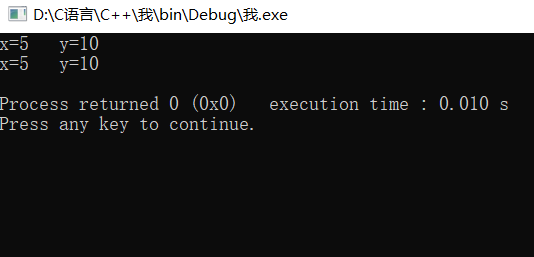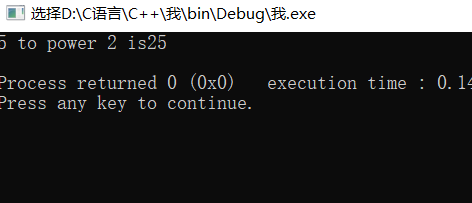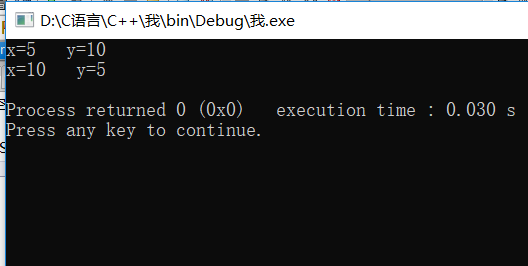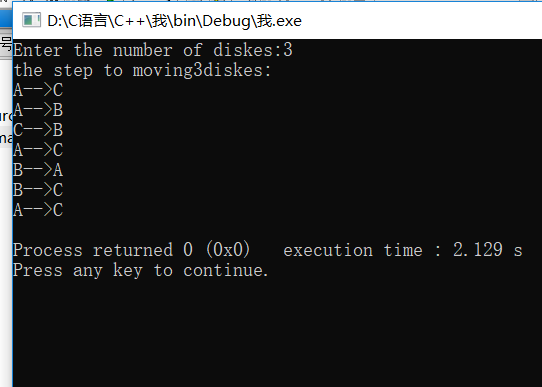## C++第二次作业

2019-09-16 21:06  jormungandlalala  阅读(119)  评论(0编辑  收藏  举报

C++第二次作业

# 教程

1.为什么要使用函数(函数编写好以后，可以被反复使用，使用时只关心函数的功能与使用方法，不必关心函数的具体实现，这样有利于代码重写，提高开发效率)

#include<iostream>
using namespace std;
double power(double x,int n){
double val=1.0;
while(n--)
val*=x;
return val;
}
int main()
{
cout<<"5 to power 2 is"<<power(5,2)<<endl;
}2.为什么要用函数重载（方便使用，便于记忆，因为C++允许功能相近的函数在相同的作用域内相同的函数名定义）

3.值传递与地址传递（函数的参数传递就是形参与实参相结合的过程，形式结合的方式有值传递与地址传递）实验P78页例3-11

#include<iostream>
using namespace std;
void swap(int a,int b){
int t=a;
a=b;
b=t;
}
int main()
{
int x=5,y=10;
cout<<"x="<<x<<"   y="<<y<<endl;
swap(x,y);
cout<<"x="<<x<<"   y="<<y<<endl;
return 0;
}#include<iostream>
using namespace std;
void swap(int &a,int &b){
int t=a;
a=b;
b=t;
}4.使用递归函数

#include<iostream>
using namespace std;
void move(char src,char dest){
cout<<src<<"-->"<<dest<<endl;
}
void hanoi(int n,char src,char medium,char dest){
if(n==1)
move(src,dest);
else{
hanoi(n-1,src,dest,medium);//以C为媒介A->B(n-1)
move(src,dest);//移最后一个盘子
hanoi(n-1,medium,src,dest);//以A为媒介B->C(n-1)
}
}
int main()
{
int m;
cout<<"Enter the number of diskes:";
cin>>m;
cout<<"the step to moving"<<m<<"diskes:"<<endl;
hanoi(m,'A','B','C');
return 0;
}